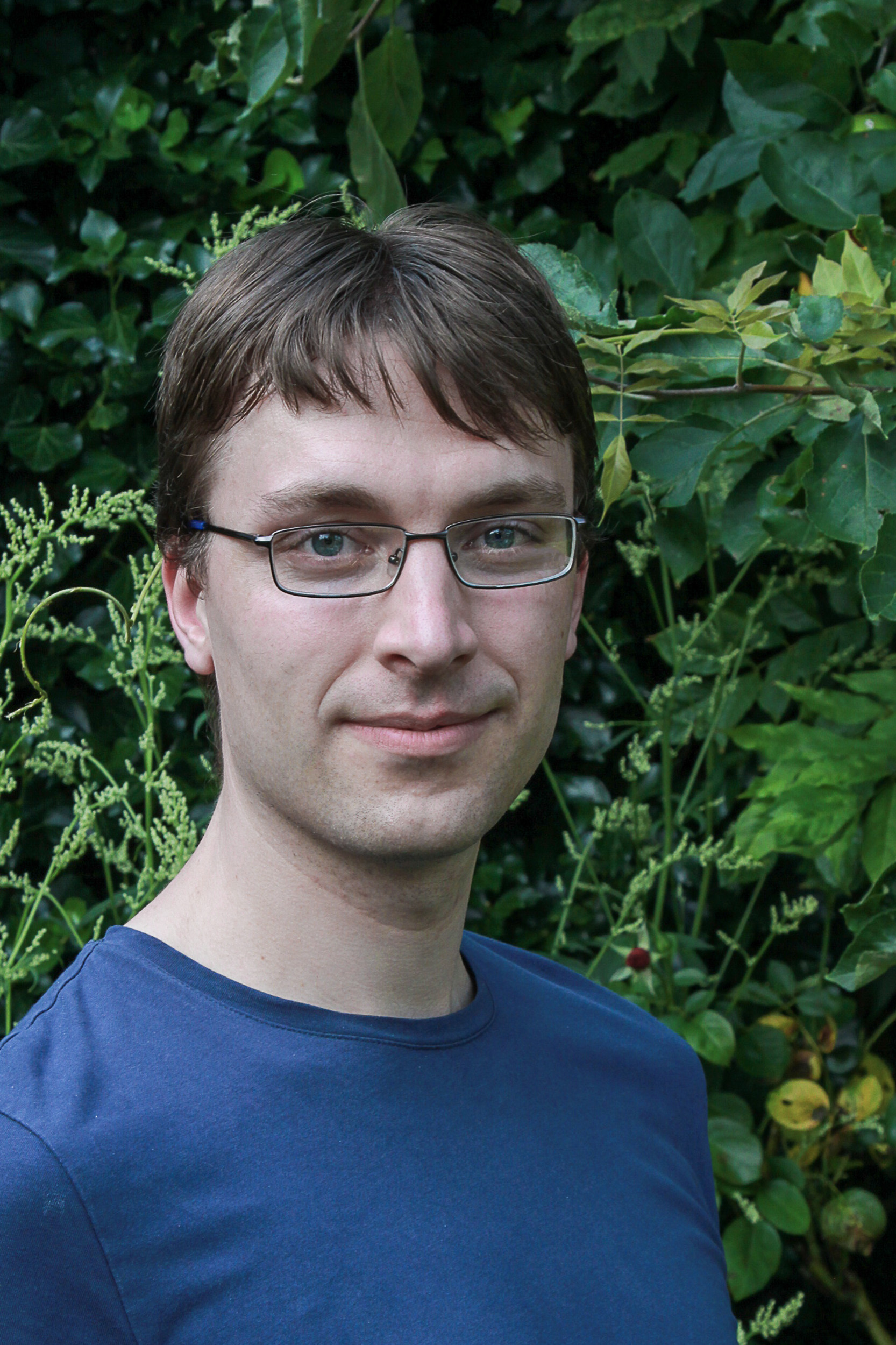# Floris van Doorn

e-mail: fpvdoorn@gmail.com

I am a postdoc at the mathematics department of the University of Paris-Saclay, working with Patrick Massot working on formalized mathematics in the Lean Theorem Prover. I am a maintainer of its mathematical library mathlib, and currently my main project is the sphere eversion project.

Until summer 2021, I was a postdoc at the mathematics department of the University of Pittsburgh, working with Tom Hales on the Formal Abstracts and Flypitch projects. I did my PhD at Carnegie Mellon University under supervision of Jeremy Avigad and Steve Awodey. My dissertation is titled On the Formalization of Higher Inductive Types and Synthetic Homotopy Theory. Full CV (pdf).

## Research

I formalize mathematical results and develop new tools for the Lean Theorem prover. I am a contributor an maintainer of mathlib. I have formalized results from many areas of mathematics, including measure theory, set theory, model theory and combinatorics. Together with Jesse Michael Han I formalized the independent of the continuum hypothesis in the Flypitch project, which was one of the few remaining unformalized problems on Freek Wiedijk's list. The proof required formalizing an internal model of set theory in Lean, and formalizing a notion of forcing. We used Boolean-valued forcing for our proof.

I also formalized another problem on Wiedijk's list, proving that it is impossible to partition a cube into finitely many smaller cubes. Recently I have formalized quite some measure theory, including the Haar measure and Fubini's theorem for the Bochner integral. I have furthermore worked on various tactics, tools and programs to aid formalization in Lean.

I am interested in Homotopy Type Theory (HoTT), which is a connection between homotopy theory and type theory, where one interprets the basic concepts in the logic of type theory in a geometric way. Using this interpretation you can define many concepts of algebraic topology, like homotopy and (co)homology groups synthetically into the type theory. Since the homotopy theoretic definitions are very close to the logical foundations, it is feasible to formalize many of these results in a proof assistant, without being much more effort than proving these results on paper. I have formalized a lot of Homotopy Type Theory in the HoTT library for Lean 2 and in the Spectral Sequence project, I was the main author of both. My main interest in this field is synthetic homotopy theory, which is the study of types as spaces in algebraic topology. My main contribution was to give a synthetic defintion of the (cohomological) Serre and Atiyah-Hirzebruch Spectral sequences in Lean. I have also studied the research question of which higher inductive types can be constructed from ordinary inductive types and one simple higher inductive type, the homotopy pushout.

## Selected Talks

Talks corresponding to one of my papers are listed under Publications.

## Teaching

University of Pittsburgh
• Spring 2021: Abstract Algebra (Math 1250).
• Spring 2020: Topics in Geometry (Math 1290).
• Spring 2019: Calculus I (Math 0220).
Carnegie Mellon University
Utrecht University